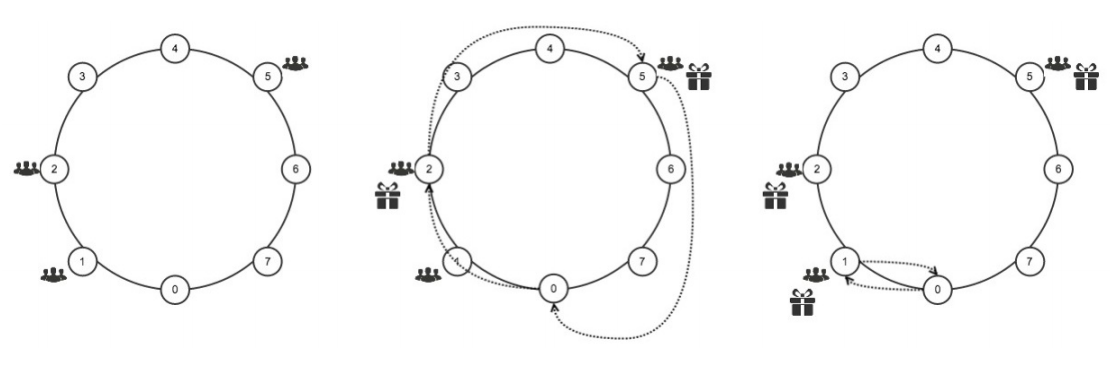# #229. 【IOI2015】Boxes

IOI2015 开幕式正在进行最后一个环节。按计划，在开幕式期间，每个代表队都将收到由主办方发放的一个装有纪念品的盒子。然而所有志愿者都被精彩的开幕式所吸引，除 Aman 以外其他人完全忘记了发放纪念品这件事。Aman 是一位热情的志愿者，为使得 IOI 尽量圆满，他要用最短的时间将所有纪念品发放出去。

Aman 可以花费 $1$ 秒的时间从一个区域移动到与他相邻的区域(顺时针或者逆时针都可以)，当 Aman 经过区域 $0$ 时他可以拿走若干纪念品，拿走纪念品是不需要时间的。任意时刻，Aman 身上只能携带不超过 $K$ 个纪念品，Aman 可以给所在区域的队伍发纪念品，这也是不需要时间的。

### 样例### 任务

• delivery(N, K, L, positions)
• N：代表队的数目
• K：Aman每次最多能携带的纪念品数量。
• L：开幕式场地上的区域数目。
• positions：一个长度为 N 的数组，positions,positions...positions[N-1]给出了所有代表队所在的区域的编号，positions的元素按非递减排序。
• 该函数应当返回 Aman 所需要的最短时间（秒数）。

### 子任务

110$1 \leq N \leq 1000$$K=1$$1 \leq L \leq 10^9$
210$K = N$
315$1 \leq N \leq 10$$1 \leq K \leq N 4151 \leq N \leq 1000 5201 \leq N \leq 10^6$$1 \leq K \leq 3000$
630$1 \leq N \leq 10^7$$1 \leq K \leq N$

### 实现细节

long long delivery(int N, int K, int L, int positions[]);

### 评测方式

1. 第 $1$ 行：$N$ $K$ $L$
2. 第 $2$ 行：$positions ... positions[N-1]$